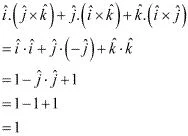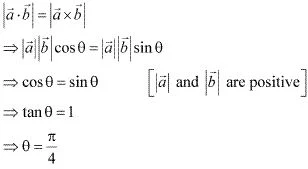# Class 12 Maths NCERT Solutions for Chapter 10 Vector Algebra Miscellaneous Exercise### Vector Algebra Miscellaneous Exercise Solutions

1. Write down a unit vector in XY-plane, making an angle of 30° with the positive direction of x-axis.

Solution

If  r is a unit vector in the XY-plane, then  r = cosθi + sinθj ^   .
Here, θ is the angle made by the unit vector with the positive direction of the x-axis.
Therefore, for θ = 30° .
r = cosθi + sinθj ^ = (√3/2)i + (1/2)j ^
Hence, the required unit vector is (√3/2) i + (1/2)j ^.

2. Find the scalar components and magnitude of the vector joining the points P(x1, y1, z1 ) and Q(x2, y2, z2) .

Solution

The vector joining the points P(x1, y1, z1) and Q(x2, y2, z2) can be obtained by,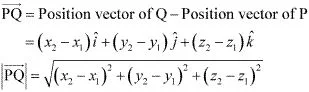Hence, the scalar components and the magnitude of the vector joining the given points are respectively {(x2 - x1), (y2 - y1), (z2 - z1)}
and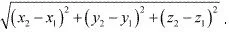3. A girl walks 4 km towards west, then she walks 3 km in a direction 30° east of north and stops. Determine the girl’s displacement from her initial point of departure.

Solution

Let O and B be the initial and final positions of the girl respectively.
Then, the girl's position can be shown as :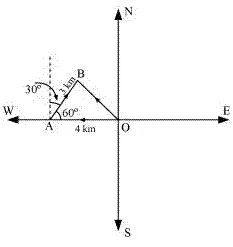Now, we have: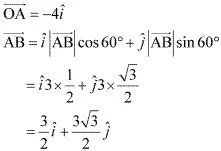By the triangle law of vector addition, we have :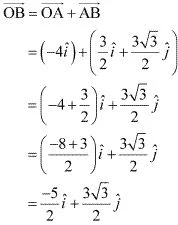Hence, the girl's displacement from her initial point of departure is (-5/2) i ^ + (3√3/2) j ^

4. If a⃗ = b⃗ + c, then is it true that |a| = |b| + |c| ? Justify your answer.
Solution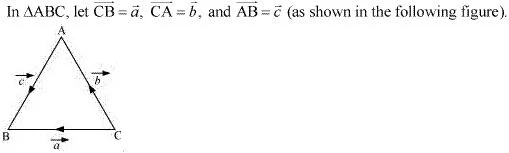Now, by the triangle law of vector addition, we have a = b⃗ + c
It is clearly known that | a|, |b|, and |c| represent the sides of ΔABC.
Also, it is known that the sum of the lengths of any two sides of a triangle is greater than the third side.
∴ | a| < |b| + |c|
Hence, it is not true that |a| = |b| + |c| .

5. Find the value of x for which x(i ^+ j ^+ k ^) is a unit vector.
Solution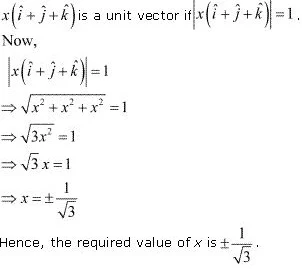6. Find a vector of magnitude 5 units, and parallel to the resultant of the vectors a = 2i ^+3j ^-k^ and b = i ^- 2j ^+ k^ .
Solution
We have,
a = 2i + 3j ^ - k^  and b = i - 2j ^ + k^
Let c be the resultant of a and b
Then,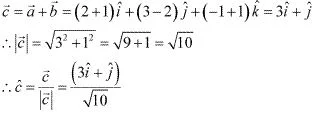Hence, the vector of magnitude 5 units and parallel to the resultant of vectors    a and  b  is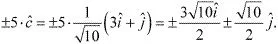7. If a = i ^+ j ^+ k^, b = 2i ^- j ^+3k^ and c = i ^- 2j ^+k^ find a unit vector parallel to the vector 2a - b +3c .
Solution
We have,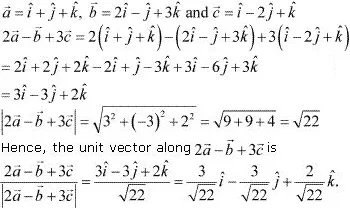8. Show that the points A (1, –2, –8), B (5, 0, –2) and C (11, 3, 7) are collinear, and find the ratio in which B divides AC.
Solution
The given points are A (1, –2, –8), B (5, 0, –2) and C (11, 3, 7) .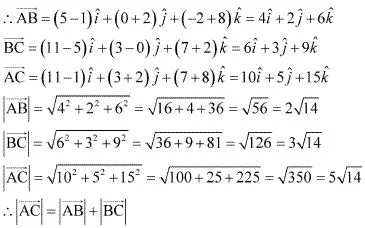Thus, the given points A, B, and C are collinear.
Now, let point B divide AC in the ratio λ : 1. Then, we have :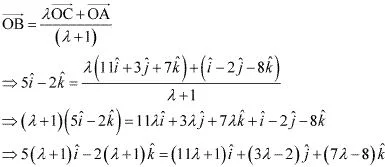On equating the corresponding  components, we get :
5(λ + 1) = 11λ + 1
⇒ 5λ + 5 =11λ + 1
⇒ 6λ = 4
⇒ λ = 4/6  = 2/3
Hence, point B divides AC in the ratio 2 : 3.

9. Find the position vector of a point R which divides the line joining two points P and Q whose position vectors are P(2a + b) and Q(a - 3b) externally in the ratio 1 : 2. Also, show that P is the mid point of the line segment RQ.
Solution
It is given that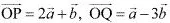It is given that point R divides a line segment joining two points P and Q externally in the ratio 1 : 2. Then, on using the section formula, we get :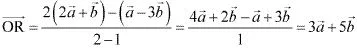Therefore, the position vector of point R is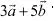Position vector of the mid - point of RQ =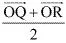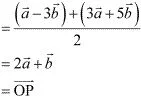Hence, P is the mid - point of the line segment RQ.

10 . The two adjacent sides of a parallelogram are 2i - 4j ^ + 5k^, and i ^-2j ^-3k^ . Find the unit vector parallel to its diagonal. Also, find its area.
Solution
Adjacent sides of a parallelogram are given as: a = 2i -4j ^+5k^  and b = i ^-2j ^+3k^  .
Then, the diagonal of a parallelogram is given by  a +  b .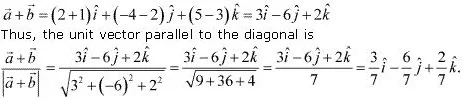∴ Area of parallelogram ABCD = | a + b |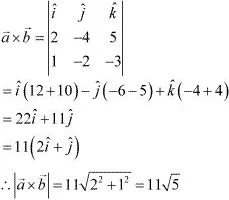Hence, the area of the parallelogram is 11√5 square units.

11. Show that the direction cosines of a vector equally inclined to the axes OX, OY, and OZ are 1/√3, 1/√3, 1/√3 .
Solution
Let a vector be equally inclined to axes OX, OY, and OZ at angle α.
Then, the direction cosines of the vector are cos α, cos α and cos α.
Now,
cos2 α + cos2 α + cos2 α = 1
⇒ 3cos2 α = 1
⇒ cos α  = 1/√3
Hence, the direction cosines of the vector which are equally inclined to the axes are 1/√3, 1/√3, 1/√3.

12. Let a = i ^+4j ^+2k^ , b=3i ^-2j ^+7k^  and c = 2i ^- j ^+4k. Find a vector d which is perpendicular to both a and b, and c . d = 15.
Solution
Let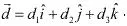Since d  is perpendicular to both a and b, we have :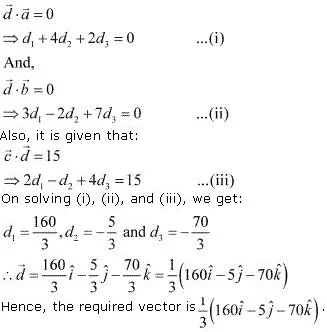13. The scalar product of the vector i ^+ j ^+ k^, with a unit vector along the sum of vectors 2i ^+4j ^-5k^ and λi ^+2j ^+3k^ is equal to one. Find the value of λ .
Solution
Hence, the value of λ is 1.

14. If  a, b, c are mutually perpendicular vectors of equal magnitudes, show that the vector a + b + c  is equally inclined to a, b and c .

Solution

Since, a , b and c  are mutually perpendicular vectors, we have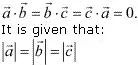Let vector a+b +c be inclined to a, b and c at angles θ1, θ2, θ3 respectively .
Then , we have :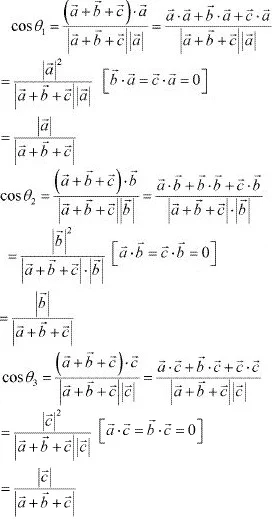Now, as |a|+|b|+ |c|, cos θ1 = cos θ2 = cosθ3.
∴  θ1 =  θ2 = θ3
Hence, the vector (a +b + c) is equally inclined to a, b, and c

15. Prove that (a +b) . (a +b) = |a|2 +|b|2 if and only if a⃗ . b are perpendicular, given a ≠ 0 , b ≠ 0
Solution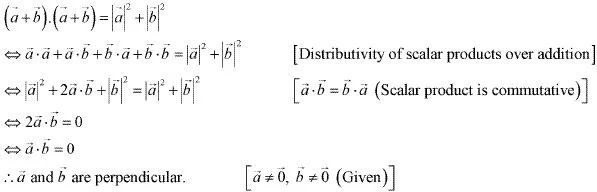16. If θ is the angle between two vectors a and b, then a . b ≥ 0 only when
(A) 0 < θ < π/2
(B) 0 ≤ θ ≤ π/2
(C)  0 < θ < π
(D)  0 ≤ θ ≤ π
Solution
Let θ be the angle between two vectors  a and b .
Then, without loss of generality, a and b are non-zero vectors so that |a| and |b| are positive.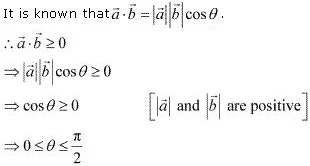Hence, a . b  ≥ 0 when 0 ≤ θ ≤ π/2  .

17. Let  a and  b  be two unit vectors and θ is the angle between them. Then a + b is a unit vector if ____
(A) θ = π/4
(B) θ = π/3
(C) θ = π/2
(D) θ = 2π/3
Solution
Let a and b be two unit vectors and θ be the angle between them.
Then, | a| = |b| = 1.
Now, a + b is a unit vector if |a +b| = 1.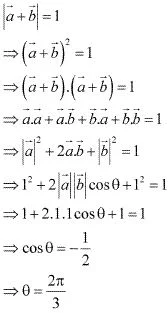Hence, a + b is a unit vector if θ = 2π/3.

18.  The value of is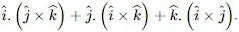(A) 0
(B) -1
(C) 1
(D) 3
Solution